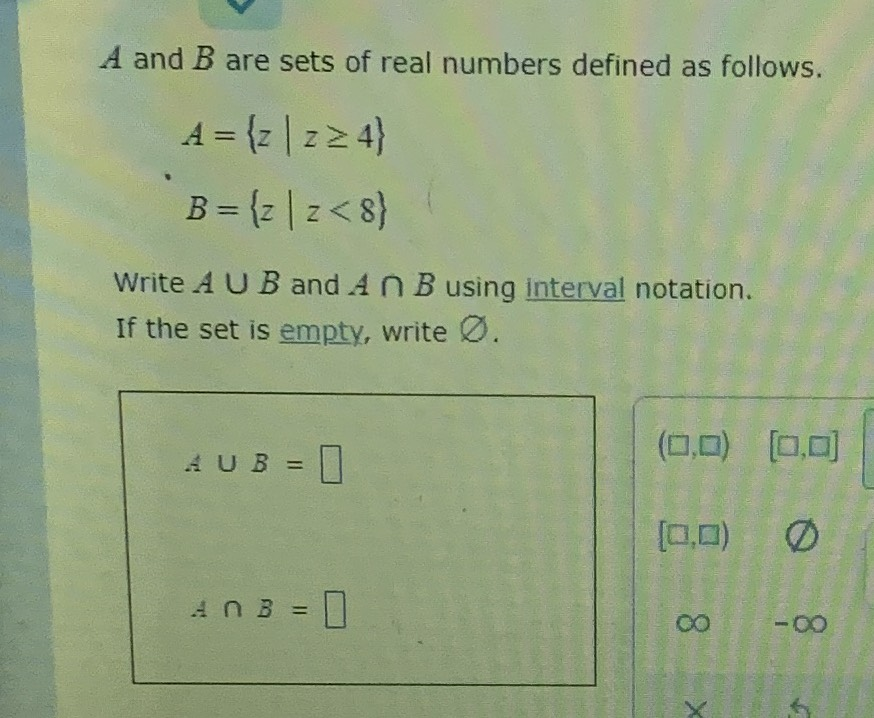### ¿Todavía tienes preguntas de matemáticas?

Pregunte a nuestros tutores expertos
Algebra
Pregunta$$A$$ and $$B$$ are sets of real numbers defined as follows. $$A = \{ z | z \geq 4 \}$$ $$B = \{ z | z < 8 \}$$

Write $$A \cup B$$ and $$A \cap B$$ using interval notation. If the set is empty, write $$\varnothing$$ .

$$A \cup B = \square$$

$$A \cap B = \square$$

$$A\cup B= (- \infty ,\infty )\\A\cap B= [4,8)$$